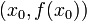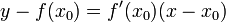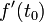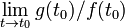# Tangent line

## Definition

### For a curve

The tangent line to a curve at a point is the best local straight line appropximation to the curve at the point.

### For the graph of a function

Suppose$f$ is the graph of a function of one variable and$x_0$ is a point in the domain of$f$ such that$f$ is continuous at$x_0$. The tangent line through the point$(x_0,f(x_0))$ to the graph of$f$ is defined as follows:

• If the derivative$f'(x_0)$ exists and is finite, it is given by the equation:$\! y - f(x_0) = f'(x_0)(x - x_0)$

• If both the one-sided limits for the derivative give the same sign of infinity (i.e., either both give$+\infty$ or both give$-\infty$) then we say we have a vertical tangent and the equation is:$\! x = x_0$

• If neither of the above are true, there is no well defined tangent line through the point.

### For a parametric description

See parametric differentiation for more.

Consider a curve described parametrically by$x = f(t), y = g(t)$. At$t = t_0$, the tangent line (geometrically, to the point$(f(t_0),g(t_0))$), is defined as follows:

• If the derivatives$f'(t_0)$ and$g'(t_0)$ exist as finite numbers and are not both zero:$\! f'(t_0)(y - g(t_0)) = g'(t_0)(x - f(t_0))$

• If both the derivatives are zero, then we try to see if$\lim_{t \to t_0} g(t_0)/f(t_0)$ exists and is finite, and if so, take that as the derivative that we plug into the equation for the tangent line. If it is a single signed infinity, we get a vertical tangent.